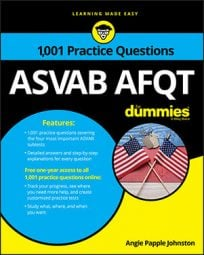##### ASVAB AFQTProblems that ask you to compare amounts are pretty common on the Arithmetic Reasoning subtest on the ASVAB. These questions have two things in common: they often look trickier than they actually are, and they can all be solved with a little algebra.

## Practice questions

1. Kim has a pocket full of change that includes pennies, nickels, and dimes. She has three more nickels than dimes and four times as many pennies as nickels. How many of each coin does Kim have if the total value is 65 cents?

A. 2 dimes, 5 nickels, and 20 pennies B. 4 dimes, 3 nickels, and 16 pennies C. 4 dimes, 6 nickels, and 1 penny D. 5 dimes, 2 nickels, and 1 penny

2. Queiana and Charles are choosing teams for dodgeball. There are b boys in the class, which is two more than twice the number of girls in the class. How many girls are in the class?

1. The correct answer is Choice (A).

The best way to solve this problem is to create a simple chart, with one column showing the number of each coin and another column showing the monetary value. Don't worry — you're allowed to use as much scratch paper as you need when you take the ASVAB. Let x represent the number of dimes Kim has, and let x + 3 represent the number of nickels she has. Finally, let 4(x + 3) equal the number of pennies she has. Your chart should look something like this:

Kim has a total of 65 cents, so add the values in cents and set that equal to 65. Now you can solve for x, the number of dimes:

Now that you know x = 2, you can determine that Kim has 2 dimes, 5 nickels, and 16 pennies in her pocket. Check your math by adding up the monetary value of her coins:

\$0.20 + \$0.25 + \$0.20 = \$0.65

2. The correct answer is Choice (A).

The problem tells you that the number of boys is b, and you can let x be the number of girls. The number of boys is two more than twice the number of girls, so let 2x + 2 represent the number of boys. That means b = 2x + 2.

You can express this relationship in an equation, and as with any equation, you need to isolate the variable you want to find. Rearrange the equation to get x by itself: